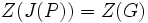# Glauberman type not implies p-constrained

This article gives the statement and possibly, proof, of a non-implication relation between two group properties. That is, it states that every group satisfying the first group property (i.e., group of Glauberman type for a prime) need not satisfy the second group property (i.e., p-constrained group)
View a complete list of group property non-implications | View a complete list of group property implications
Get more facts about group of Glauberman type for a prime|Get more facts about p-constrained group

## Statement

It is possible to have a prime number$p$ and a finite group$G$ such that$G$ is a group of Glauberman type for$p$ but is not a p-constrained group.

## Proof

### Example of the special linear group

Further information: special linear group:SL(2,5), subgroup structure of special linear group:SL(2,5)

Let$G = SL(2,5)$ and$p = 2$. Then,$O_{p',p}(G) = Z(G)$ is cyclic of order two, and$G/Z(G)$ is a simple group isomorphic to alternating group:A5. If$P$ is a$2$-Sylow subgroup of$G$, then$P$ is isomorphic to a quaternion group and$P \cap O_{p',p}(G) = Z(G)$, so$C_G(P \cap O_{p',p}(G)) = G \not \subseteq O_{p',p}(G)$. Thus,$G$ is not$p$-constrained.

On the other hand,$Z(J(P)) = Z(G)$, so$O_{p'}(G)N_G(Z(J(P))) = N_G(Z(G)) = G$, so$G$ is of Glauberman type with respect to the prime$p = 2$.